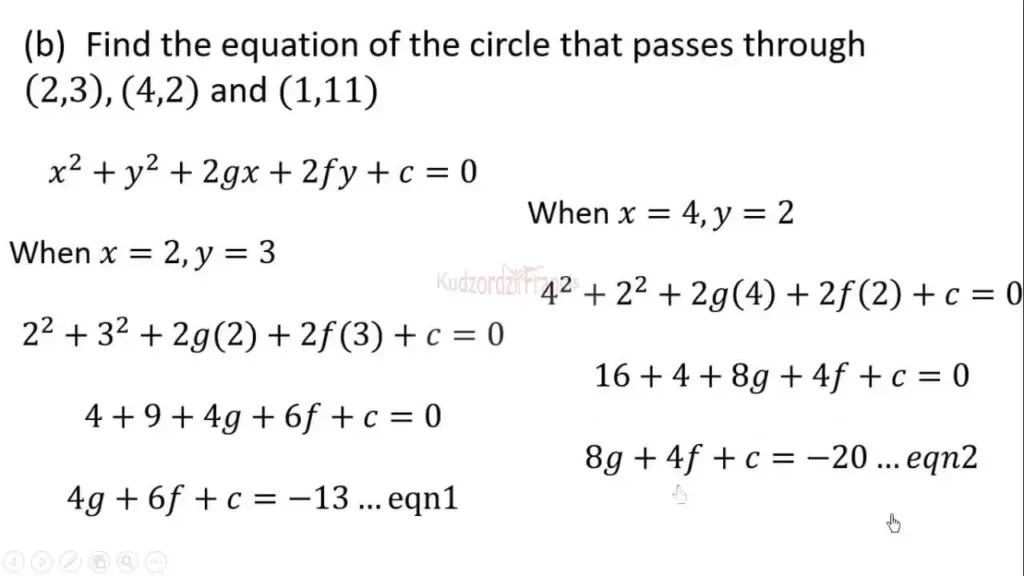# WASSCE 2023 Elective Mathematics Questions & Final Projected TopicsWASSCE 2023 Elective Mathematics questions

## The 2023 WASSCE Elective Mathematics Questions (paper) is scheduled to start today, 5th September 2023. Here are the final-confirmed topics and sample questions to try.

The WASSCE 2023 Elective Mathematics Questions will be administered by WAEC today. The Elective Mathematics paper will be written by all General Science students, some business students, and some General Arts students.

The Elective Mathematics paper will be in two sessions, the first session will be the theory part, thus, the section B and the second and last session will be the objectives or Section A.

Here are the final and confirmed topics for the 2023 WASSCE Elective Mathematics Questions;

### Final Projected Questions For 2023 Elective Mathematics

1. A ball is thrown vertically upwards. Its height (h) metres at time (t) seconds is given by h = 5 + 30t – 5t ^ 2 Find the maximum height reached by the ball.

2. A committee of 5 men and 3 women is to be selected from 10 men and 8 women. In how many ways can this be done if a particular woman must be in the committee?

3. A non-uniform beam, PQ, of weight 160 N, is 100 cm long. It is kept in a horizontal position by two vertical strings attached at points 10 cm and 15 cm from P and Q respectively. The centre of gravity of the beam acts at a distance of 45 cm from P. If a weight of 180N is hung at a distance of 35 cm from Q, calculate the tension in each string.

5. Given that u = 4i – 4j and v = 3i + 3j, find the unit vector in the direction of 3u + 2v.

6. In a particular hostel, 60% of the students use Facebook, 40% use WhatsApp and 20% use both. If a student is selected at random from the hostel, find the probability that the student uses:

• (i) Neither Facebook nor WhatsApp:
• (ii) only one of them.

7. In how many ways can 6 players be lined up if 2 particular players must not stand next to each other?

8. A bag contains 4 green and 7 blue identical balls. Three balls are selected at random, one after the other without replacement, from the bag. Find the probability that:

• (i) One ball is green, and two balls are blue;
• (ii) two of the balls have the same colour.

9. A body of mass 3 * 10 ^ 4 / moving with velocity 15m * r ^ – 1 collides with another body of mass 2 * 10 ^ 4 kg moving with velocity 10m * s ^ – 1 If the two bodies moved together after the collision, find their common velocity if they were moving in:

• (i) the same direction;
• (ii) opposite direction, before collision.

## 10. The equation of a curve is x * y ^ 3 – 2x ^ 2 * y ^ 2 = 0 Find the gradient of the tangent to the curve at (1, 2)

READ ALSO: WASSCE 2023: Sample Government Questions For Candidates